## [JAC252] Geometry Optimization of a Solenoid Valve

Remember me

*It is different from the service for JMAG WEB MEMBER (free member). Please be careful.
About authentication ID for JMAG website

### Overview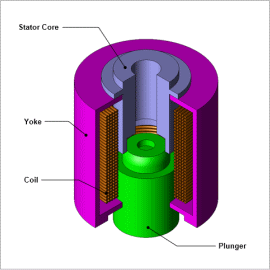A solenoid valve is used to adjust the inflow or outflow of liquids or gases by using the translational movement of its iron core. If an electric current is passed through its coil, an electromagnet is created and an electromagnetic attractive force is generated between the mover and fixed parts. Since high responsiveness is required for valve opening and closing, an important item to evaluate is whether or not the power source used for driving and the solenoid valve meets the required responsiveness and required attractive force.
In order for a solenoid valve to function well, it is necessary to improve control signal responsiveness. To this end, it is necessary to shorten the displacement time of the mover. However, since the degree of freedom in the structure of a solenoid valve can be increased, there is a demand to minimize the volume of the solenoid valve. In order to satisfy these conditions, applying automatic processing using an FEA optimization function can greatly reduce man-hours.
In this example, an example where the geometry of a solenoid valve is optimized using a multi-objective optimization algorithm is presented.

### Optimization Conditions

The mover displacement time is the measured time from when the coil is energized until the mover reaches its final position. Therefore, the influence of the mover geometry on the displacement time is focused on.
Fig. 1 shows the geometry parameters which are variables. Geometry parameters for optimization can be represented by two parameters, the radius and a notch coefficient. Table 1 shows equations for parameters showing the relationship between geometry parameters.
In this example there is a trade-off in that the volume becomes large when the mover displacement time is short and the mover displacement time becomes long if the volume is small. Optimization is performed to shorten the displacement time and minimize the volume. Table 2 shows the optimization design variables, a constraint condition, and the objective functions.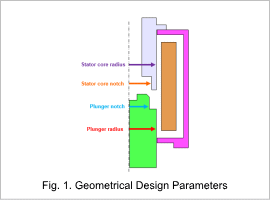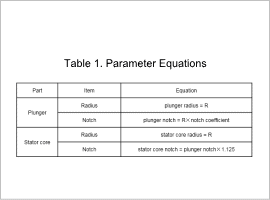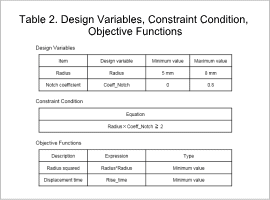### Displacement time and volume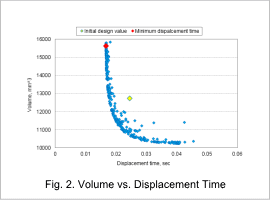Fig. 2 shows the volume vs. displacement time graph. It is assumed that the plunger reaches the drive range upper limit (final position) 0.06 sec or before. Cases that do not satisfy this assumption are excluded.
It can be seen that increasing the radius thus the volume shortens the time for the mover to reach its final position. The minimum displacement time is 16.8 msec, for which the radius is 7.9 mm and the volume is 15,621 mm^3.
The case for the initial design values is indicated by a yellow marker, and the case for the minimum displacement time is indicated by a red marker. Comparing the case for the minimum displacement time with the case for the initial design, for the minimum displacement time case the displacement time decreases by 32% but the volume increases by 23%.

### Volume times displacement time vs. radius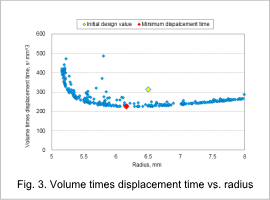In order to find the optimum value between the displacement time and the volume, the volume times displacement time (volume × displacement time) is evaluated. A graph of volume times displacement time vs. radius is shown in Fig. 3. It is assumed that the plunger reaches the drive range upper limit (final position) 0.06 sec or before. Cases that do not satisfy this assumption are excluded.
The case for the initial design values is indicated by a yellow marker, and the case for the minimum displacement time is indicated by a red marker. In Fig. 3 it can be seen that the optimized values are 224 s·mm^3 for volume times displacement time and 6.16 mm for the radius. In this case, the volume decreases by 5% from the initial design and the volume times displacement time decreases by 28%. This shows that the displacement time for a given volume improves.